Ashish Maurya

teacher | English EVS Environmental Studies Hindi Physics Chemistry

Description

Hi Myself Ashish Maurya, Teaches Physics, Chemistry, Maths, Science.

M.Sc

5-7 Years

11-12

Current Organization

U P Mahaidhyalay

Radioactive Displacement Law OR Fajan's and Soddy Law

Radioactive Displacement Law OR Fajan's and Soddy Law

It describe which chemical element and isotope is created during the perticular type of radioactive decay.

(I)  Alpha - decay :

In alpha - decay, the mass number of the product number of the product nucleus is four less than that of decaying nucleus while the atomic number decreses by two.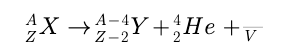(II) beta - decay :

In beta- decay, the mass number of product nucleus remains same but atomic number increases or decrease by one.

In beta -minus decay, as electron and an antinuetrino are created and emmited from the nuclues via the reaction given below :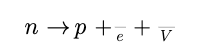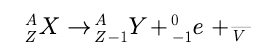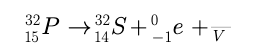In beta -plus decay, a positron and neutrino are created and emmited from nuclues via the reaction given below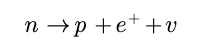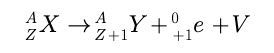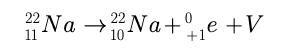gamma - decay :

A ray is emmited when alpha or beta - decay results in a nucleus in an excited state. Atom then returns to grround state by a single photon transition or succesive transitions invloving more than on photon.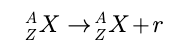Physics - Triangle Laws of Vectors

Triangle Laws of Vectors

Statement :

" If two vectors are represented in magnitude and direction by the two sides of a triangle taken in order, then the third side of the triangle taken in reverse order represents their resultant in magnitude and direction "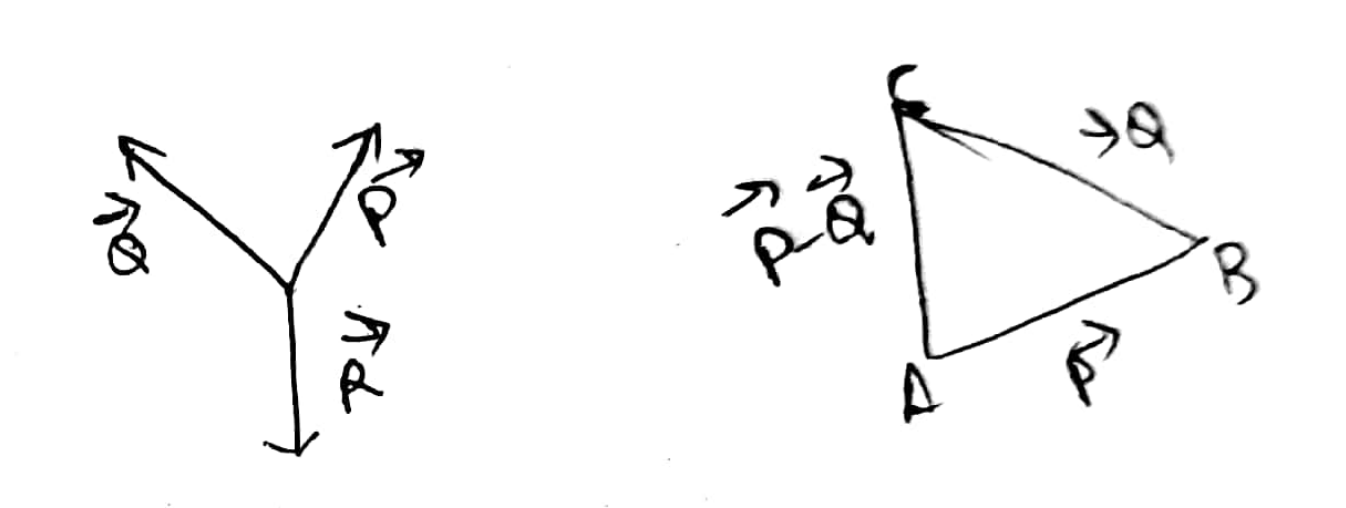Explanation :

Suppose two vectors P and Q are acting at the point Q simultaneously. P and Q are represented in both magnitude and direction by AB and BC respectively. Then the third side taken in the reverse order i.e AC represent their resultant in both magnitue and direction.

P + Q = -R

P + Q + R = 0

which means that the three vectors P , Q and R keep the pont 'O' in equilibrium.

Physics - Friction Forces - what is Friction Force ?

Friction Forces

When two bodies are kept in contact, electro-magnetic forces act between the charged particles at the surface of the bodies. As a result each body exerts a contact force on the other.

The magnitude of the contact forces on the two bodies are equal but their dirrection are opposite and hence the contact forces obey Newton's IIIrd Law.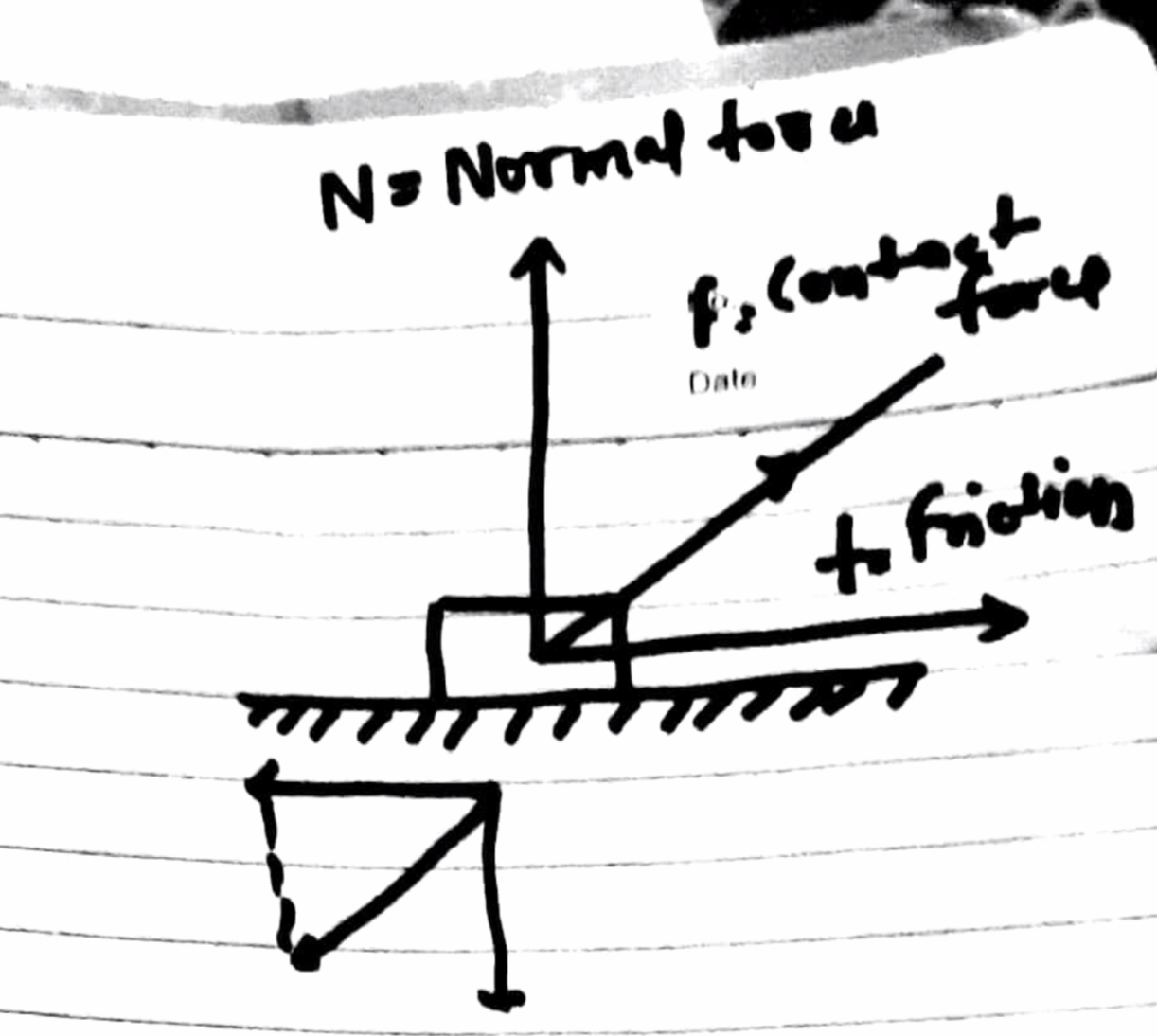Static Friction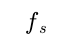When two bodies do not slip on each other the force of friction is called Static Friction